Next: Mass and weight Up: Newton's laws of motion Previous: Hooke's law

## Newton's third law of motion

Suppose, for the sake of argument, that there are only two bodies in the Universe. Let us label these bodiesand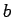. Suppose that bodyexerts a forceon body. According to to Newton's third law of motion, bodymust exert an equal and opposite forceon body. See Fig. 22. Thus, if we labelthe action'' then, in Newton's language,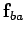is the equal and opposed reaction''.

Suppose, now, that there are many objects in the Universe (as is, indeed, the case). According to Newton's third law, if object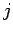exerts a force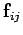on object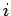then objectmust exert an equal and opposite force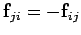on object. It follows that all of the forces acting in the Universe can ultimately be grouped into equal and opposite action-reaction pairs. Note, incidentally, that an action and its associated reaction always act on different bodies.Why do we need Newton's third law? Actually, it is almost a matter of common sense. Suppose that bodiesandconstitute an isolated system. Ifthen this system exerts a non-zero net forceon itself, without the aid of any external agency. It will, therefore, accelerate forever under its own steam. We know, from experience, that this sort of behaviour does not occur in real life. For instance, I cannot grab hold of my shoelaces and, thereby, pick myself up off the ground. In other words, I cannot self-generate a force which will spontaneously lift me into the air: I need to exert forces on other objects around me in order to achieve this. Thus, Newton's third law essentially acts as a guarantee against the absurdity of self-generated forces.Next: Mass and weight Up: Newton's laws of motion Previous: Hooke's law
Richard Fitzpatrick 2006-02-02